## Step 1: Find the least common denominators

Given a set of fractions with unlike denominators, find the least common denominator (LCD) shared by the fractions. So, if we want to arrange 1/35, 3/7, 7/10, 4/5 and 12/35 from greatest to least, let’s determine the LCD shared by the fractions first.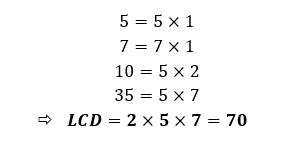## Step 2: Determine the equivalent fractions sharing the LCD

For our example, we express the fractions so that they all share a denominator of 70.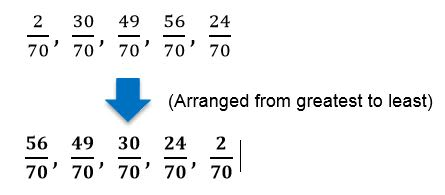## Step 3: Arrange the numerators

Arrange the numerators’ values based on the order required to arrange the fractions. So for our example, we have:## Step 4: Rewrite the fractions

Once the equivalent fractions are placed in order, rewrite the fractions back to their original given form. Hence, if the set of fractions are arranged from greatest to least, we have: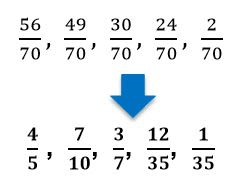## Examples of how to order fractions

Q1) Arrange the set of fractions from greatest to least.

41/96, 17/48, 11/36, 17/24

The denominators of the fractions are not the same so we first need to determine the LCD shared by the fractions.

24=12×2

36=12×3

48=12×4

96=12×4×2

LCD=12×4×3×2=288

Rewrite the fractions so that they share the same denominator: 288.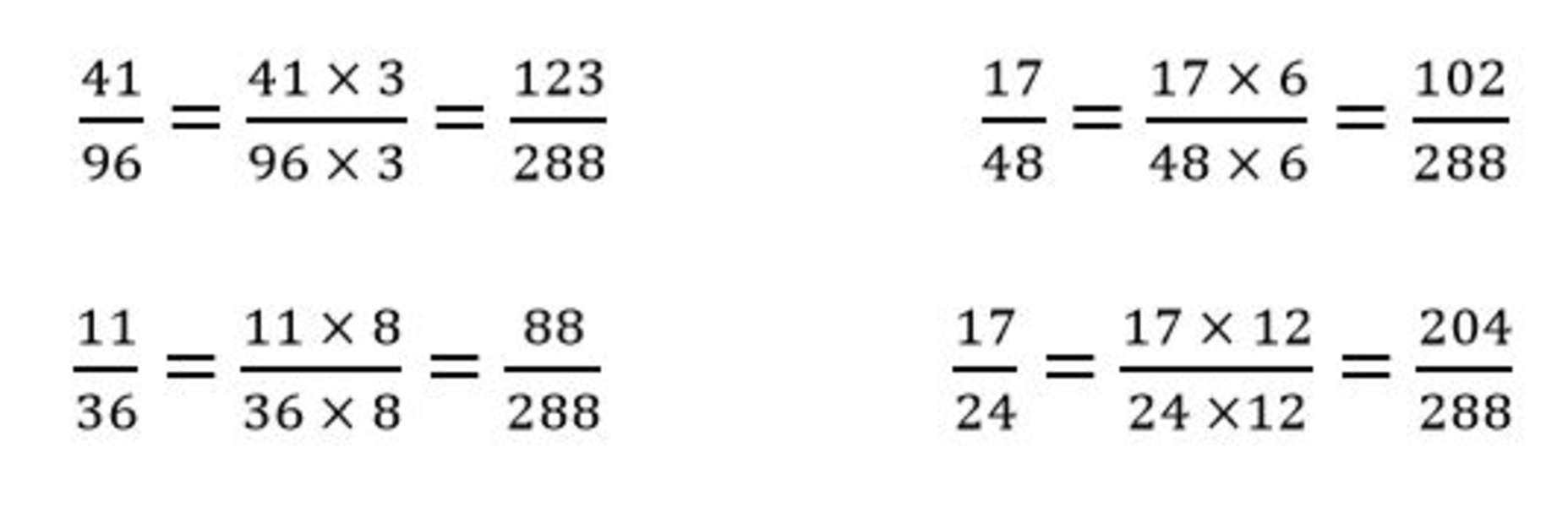Once the denominators for the four fractions are the same, we can arrange the numerators from greatest to least.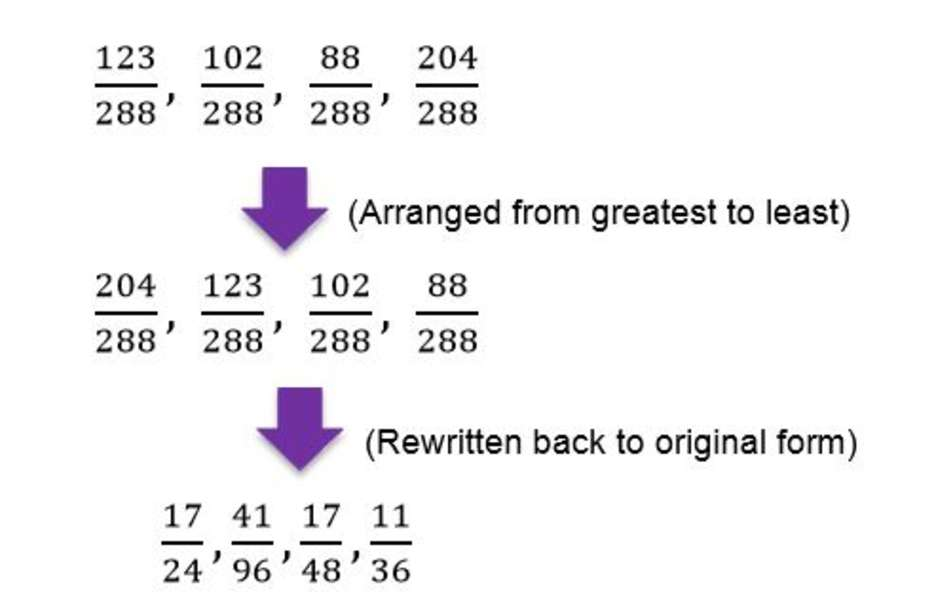After arranging the equivalent fractions, we can rewrite the fractions back to their given form. Hence, we have the following fractions arranged from greatest to least.